Next: 12.9 Some XVIII-th Century Up: 12. Power Series Previous: 12.7 Special Values of   Index

# 12.8 Proof of the Differentiation Theorem

12.73   Lemma. The power series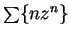has radius of convergence equal to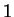.

12.74   Exercise. Prove lemma 12.73. (We proved this lemma earlier using the differentiation theorem. Since we need this result to prove the differentiation theorem, we now want a proof that does not use the differentiation theorem.)

12.75   Lemma. Let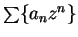be a power series. Then the two seriesand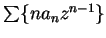have the same radius of convergence.

Proof: I'll show that for all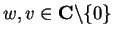.

a) If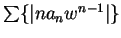converges, then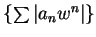converges.
b) Ifconverges and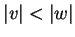, then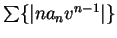converges.
a) follows from the comparison test, sinceTo prove b), supposeconverges and. By lemma 12.73,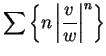converges, and henceis bounded. Choose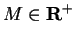such that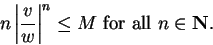Then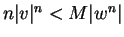, and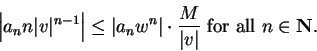By the comparison test,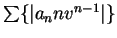converges.12.76   Corollary.and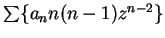have the same radius of convergence.

Proof: Use the lemma twice.12.77   Theorem. Let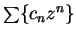be a power series with positive radius of convergence. Let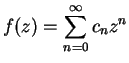for all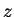in the disc of convergence for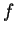and let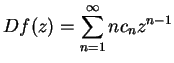be the function corresponding to the formal derivative of. Thenis differentiable on its disc of convergence, and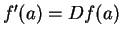for all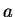in the disc of convergence.

Proof: Letbe a point in the disc of convergence, and letbe a different point in the disc. Then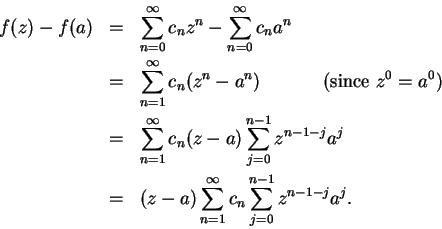Let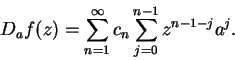Then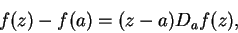and since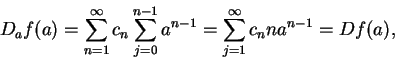the theorem will follow if we can show that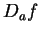is continuous at.

In the calculation below, I quietly use the following facts:

a) When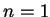,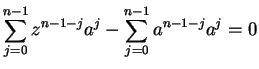.
b) When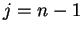,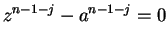.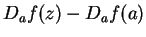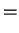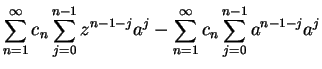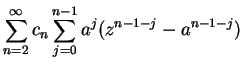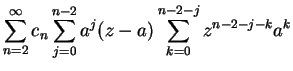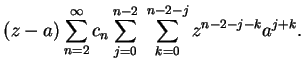(12.78)

Let the radius of convergence of our power series be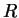, and let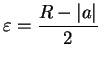. Then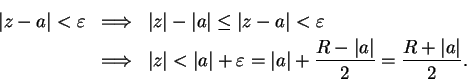Let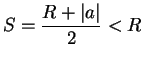. Then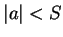, and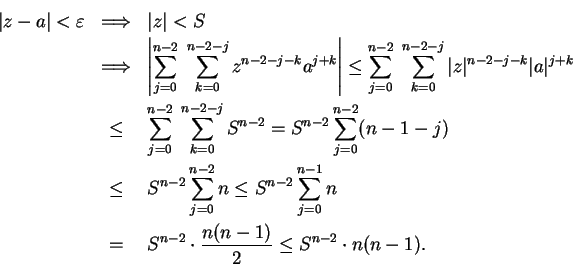(Here I've used the fact that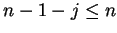for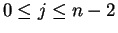.) Thus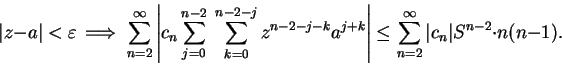We noticed in the corollary to lemma 12.75 that the series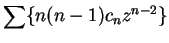has radius of convergence, and hence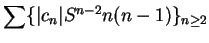converges to a limit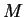, and by (12.78),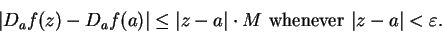If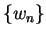is a sequence in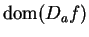such that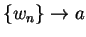, then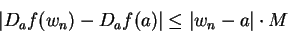for all large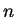, and by the null-times bounded theorem and comparison theorem for null sequences,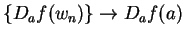. Hence,is continuous at.Next: 12.9 Some XVIII-th Century Up: 12. Power Series Previous: 12.7 Special Values of   Index